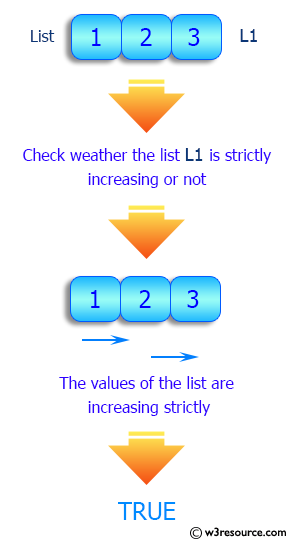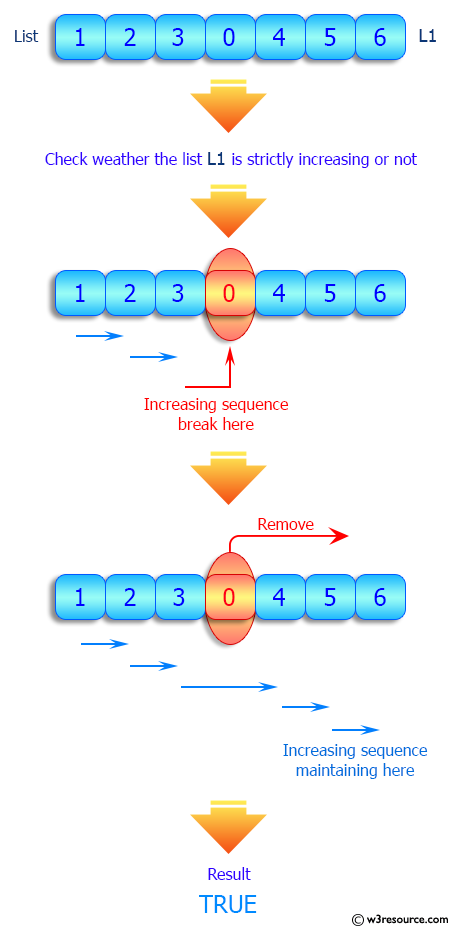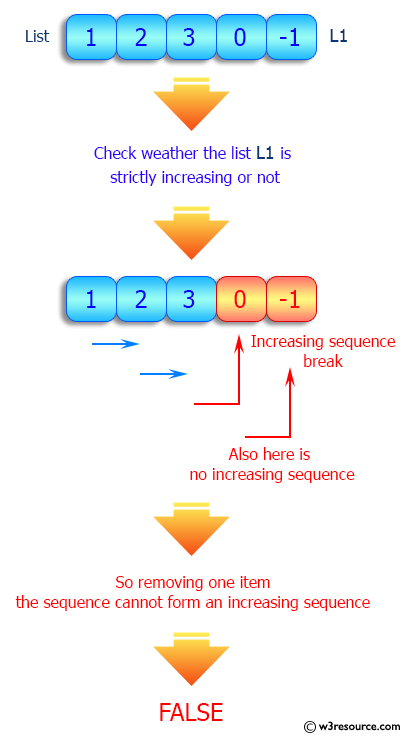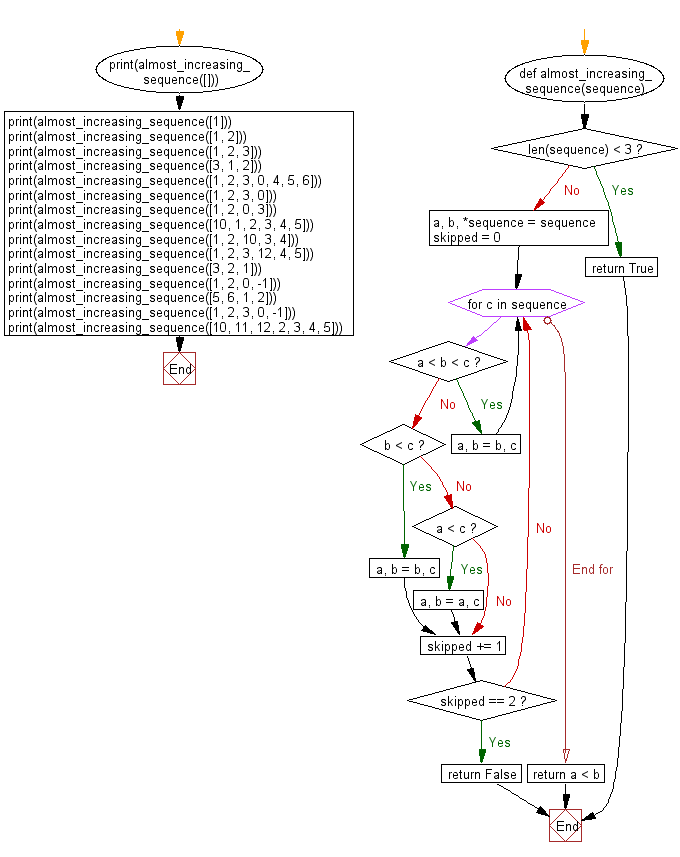﻿ Python: Check if a given list is strictly increasing or not - w3resource# Python: Check if a given list is strictly increasing or not

## Python List: Exercise - 161 with Solution

Write a Python program to check if a given list is strictly increasing or not. Moreover, If removing only one element from the list results in a strictly increasing list, we still consider the list true.

Sample Solution:

Python Code:

``````# Source: https://bit.ly/3qZqcwm
def almost_increasing_sequence(sequence):
if len(sequence) < 3:
return True

a, b, *sequence = sequence
skipped = 0
for c in sequence:
if a < b < c:  # XXX
a, b = b, c
continue
elif b < c:    # !XX
a, b = b, c
elif a < c:    # X!X
a, b = a, c
skipped += 1
if skipped == 2:
return False
return a < b

print(almost_increasing_sequence([]))
print(almost_increasing_sequence())
print(almost_increasing_sequence([1, 2]))
print(almost_increasing_sequence([1, 2, 3]))
print(almost_increasing_sequence([3, 1, 2]))
print(almost_increasing_sequence([1, 2, 3, 0, 4, 5, 6]))
print(almost_increasing_sequence([1, 2, 3, 0]))
print(almost_increasing_sequence([1, 2, 0, 3]))
print(almost_increasing_sequence([10, 1, 2, 3, 4, 5]))
print(almost_increasing_sequence([1, 2, 10, 3, 4]))
print(almost_increasing_sequence([1, 2, 3, 12, 4, 5]))

print(almost_increasing_sequence([3, 2, 1]))
print(almost_increasing_sequence([1, 2, 0, -1]))
print(almost_increasing_sequence([5, 6, 1, 2]))
print(almost_increasing_sequence([1, 2, 3, 0, -1]))
print(almost_increasing_sequence([10, 11, 12, 2, 3, 4, 5]))
```
```

Sample Output:

```True
True
True
True
True
True
True
True
True
True
True
False
False
False
False
False
```

Pictorial Presentation:Flowchart:## Visualize Python code execution:

The following tool visualize what the computer is doing step-by-step as it executes the said program:

Python Code Editor:

Have another way to solve this solution? Contribute your code (and comments) through Disqus.

What is the difficulty level of this exercise?

Test your Python skills with w3resource's quiz

﻿

## Python: Tips of the Day

Floor Division:

When we speak of division we normally mean (/) float division operator, this will give a precise result in float format with decimals.

For a rounded integer result there is (//) floor division operator in Python. Floor division will only give integer results that are round numbers.

```print(1000 // 300)
print(1000 / 300)```

Output:

```3
3.3333333333333335```